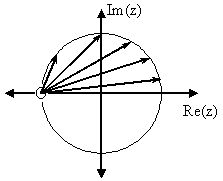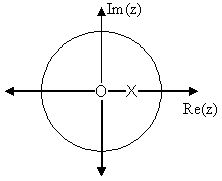# 12.8 Discrete time filter design

 Page 1 / 1
Describes how to design a general filter from the Z-Transform and it pole/zero plots.

## Estimating frequency response from z-plane

One of the primary motivating factors for utilizing the z-transform and analyzing the pole/zero plots is due to their relationship to the frequency responseof a discrete-time system. Based on the position of the poles and zeros, one can quickly determine the frequency response. This is aresult of the correspondence between the frequency response and the transfer function evaluated on the unit circle in thepole/zero plots. The frequency response, or DTFT, of the system is defined as:

$H(w)=(z, e^{iw}, H(z))=\frac{\sum_{k=0}^{M} {b}_{k}e^{-(iwk)}}{\sum_{k=0}^{N} {a}_{k}e^{-(iwk)}}$
Next, by factoring the transfer function into poles and zeros and multiplying the numerator and denominator by $e^{iw}$ we arrive at the following equations:
$H(w)=\left|\frac{{b}_{0}}{{a}_{0}}\right|\frac{\prod_{k=1}^{M} \left|e^{iw}-{c}_{k}()\right|}{\prod_{k=1}^{N} \left|e^{iw}-{d}_{k}()\right|}$
From [link] we have the frequency response in a form that can be used to interpretphysical characteristics about the filter's frequency response. The numerator and denominator contain a product ofterms of the form $\left|e^{iw}-h\right|$ , where $h$ is either a zero, denoted by ${c}_{k}$ or a pole, denoted by ${d}_{k}$ . Vectors are commonly used to represent the term and its parts on the complex plane. The pole or zero, $h$ , is a vector from the origin to its location anywhere on the complex plane and $e^{iw}$ is a vector from the origin to its location on the unit circle. The vector connecting these twopoints, $\left|e^{iw}-h\right|$ , connects the pole or zero location to a place on the unit circle dependent on the value of $w$ . From this, we can begin to understand how the magnitude of the frequency response is aratio of the distances to the poles and zero present in the z-plane as $w$ goes from zero to pi. These characteristics allow us to interpret $\left|H(w)\right|$ as follows:
$\left|H(w)\right|=\left|\frac{{b}_{0}}{{a}_{0}}\right|\frac{\prod \mathrm{"distances from zeros"}}{\prod \mathrm{"distances from poles"}}$

## Drawing frequency response from pole/zero plot

Let us now look at several examples of determining the magnitude of the frequency response from the pole/zero plot ofa z-transform. If you have forgotten or are unfamiliar with pole/zero plots, please refer back to the Pole/Zero Plots module.

In this first example we will take a look at the very simple z-transform shown below: $H(z)=z+1=1+z^{-1}$ $H(w)=1+e^{-(iw)}$ For this example, some of the vectors represented by $\left|e^{iw}-h\right|$ , for random values of $w$ , are explicitly drawn onto the complex plane shown in the figure below. These vectors show how the amplitude of the frequency response changes as $w$ goes from $0$ to $2\pi$ , and also show the physical meaning of the terms in [link] above. One can see that when $w=0$ , the vector is the longest and thus the frequency responsewill have its largest amplitude here. As $w$ approaches $\pi$ , the length of the vectors decrease as does the amplitude of $\left|H(w)\right|$ . Since there are no poles in the transform, there is only this onevector term rather than a ratio as seen in [link] .The first figure represents the pole/zero plot with a few representative vectors graphed while the second shows thefrequency response with a peak at +2 and graphed between plus and minus .

For this example, a more complex transfer function is analyzed in order to represent the system's frequencyresponse. $H(z)=\frac{z}{z-\frac{1}{2}}=\frac{1}{1-\frac{1}{2}z^{-1}}$ $H(w)=\frac{1}{1-\frac{1}{2}e^{-(iw)}}$

Below we can see the two figures described by the above equations. The [link] represents the basic pole/zero plot of the z-transform, $H(w)$ . [link] shows the magnitude of the frequency response. From the formulas and statements in the previoussection, we can see that when $w=0$ the frequency will peak since it is at this value of $w$ that the pole is closest to the unit circle. The ratio from [link] helps us see the mathematics behindthis conclusion and the relationship between the distances from the unit circle and the poles and zeros. As $w$ moves from $0$ to $\pi$ , we see how the zero begins to mask the effects of the poleand thus force the frequency response closer to $0$ .The first figure represents the pole/zero plot while the second shows the frequency response with a peak at+2 and graphed between plus and minus .

## Conclusion

In conclusion, using the distances from the unit circle to the poles and zeros, we can plot the frequency response of thesystem. As $w$ goes from $0$ to $2\pi$ , the following two properties, taken from the above equations, specify how one should draw $\left|H(w)\right|$ .

## While moving around the unit circle...

1. if close to a zero, then the magnitude is small. If a zero is on the unit circle, then the frequency response iszero at that point.
2. if close to a pole, then the magnitude is large. If a pole is on the unit circle, then the frequency responsegoes to infinity at that point.

where we get a research paper on Nano chemistry....?
nanopartical of organic/inorganic / physical chemistry , pdf / thesis / review
Ali
what are the products of Nano chemistry?
There are lots of products of nano chemistry... Like nano coatings.....carbon fiber.. And lots of others..
learn
Even nanotechnology is pretty much all about chemistry... Its the chemistry on quantum or atomic level
learn
da
no nanotechnology is also a part of physics and maths it requires angle formulas and some pressure regarding concepts
Bhagvanji
hey
Giriraj
Preparation and Applications of Nanomaterial for Drug Delivery
revolt
da
Application of nanotechnology in medicine
what is variations in raman spectra for nanomaterials
ya I also want to know the raman spectra
Bhagvanji
I only see partial conversation and what's the question here!
what about nanotechnology for water purification
please someone correct me if I'm wrong but I think one can use nanoparticles, specially silver nanoparticles for water treatment.
Damian
yes that's correct
Professor
I think
Professor
Nasa has use it in the 60's, copper as water purification in the moon travel.
Alexandre
nanocopper obvius
Alexandre
what is the stm
is there industrial application of fullrenes. What is the method to prepare fullrene on large scale.?
Rafiq
industrial application...? mmm I think on the medical side as drug carrier, but you should go deeper on your research, I may be wrong
Damian
How we are making nano material?
what is a peer
What is meant by 'nano scale'?
What is STMs full form?
LITNING
scanning tunneling microscope
Sahil
how nano science is used for hydrophobicity
Santosh
Do u think that Graphene and Fullrene fiber can be used to make Air Plane body structure the lightest and strongest. Rafiq
Rafiq
what is differents between GO and RGO?
Mahi
what is simplest way to understand the applications of nano robots used to detect the cancer affected cell of human body.? How this robot is carried to required site of body cell.? what will be the carrier material and how can be detected that correct delivery of drug is done Rafiq
Rafiq
if virus is killing to make ARTIFICIAL DNA OF GRAPHENE FOR KILLED THE VIRUS .THIS IS OUR ASSUMPTION
Anam
analytical skills graphene is prepared to kill any type viruses .
Anam
Any one who tell me about Preparation and application of Nanomaterial for drug Delivery
Hafiz
what is Nano technology ?
write examples of Nano molecule?
Bob
The nanotechnology is as new science, to scale nanometric
brayan
nanotechnology is the study, desing, synthesis, manipulation and application of materials and functional systems through control of matter at nanoscale
Damian
Is there any normative that regulates the use of silver nanoparticles?
what king of growth are you checking .?
Renato
What fields keep nano created devices from performing or assimulating ? Magnetic fields ? Are do they assimilate ?
why we need to study biomolecules, molecular biology in nanotechnology?
?
Kyle
yes I'm doing my masters in nanotechnology, we are being studying all these domains as well..
why?
what school?
Kyle
biomolecules are e building blocks of every organics and inorganic materials.
Joe
Got questions? Join the online conversation and get instant answers!By Anonymous UserBy JavaChamp TeamBy Joli JuliannaBy Hope PercleByBy JavaChamp TeamBy OpenStaxBy OpenStaxBy John GabrieliBy OpenStax